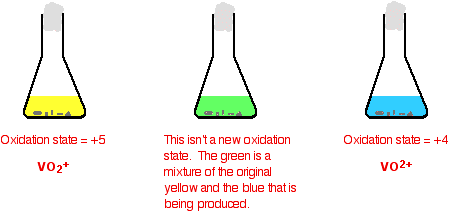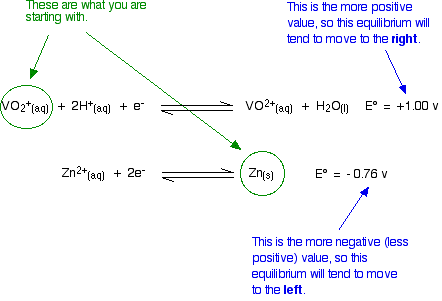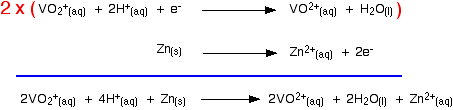Need help? Email help@shout.education

This page looks at some aspects of vanadium chemistry required for UK A-level exams. That includes the use of vanadium(V) oxide as a catalyst in the Contact Process, and converting between the various vanadium oxidation states.

## Vanadium(V) oxide as a Catalyst in the Contact Process

### The Overall Reaction

During the Contact Process for manufacturing sulfuric acid, sulfur dioxide has to be converted into sulfur trioxide. This is done by passing sulfur dioxide and oxygen over a solid vanadium(V) oxide catalyst.

\text{SO}_2 + \tfrac{1}{2}\text{O}_2 \xrightarrow{\text{V}_2\text{O}_5} \text{SO}_3

Note: The equation is written with the half in it to make the explanation below tidier. You may well be familiar with the equation written as twice that shown, but the present version is perfectly acceptable. It is also shown as a one-way rather than a reversible reaction to avoid complicating things.

### How the Reaction Works

This is a good example of the ability of transition metals and their compounds to act as catalysts because of their ability to change their oxidation state (oxidation number).

Note: If you aren't sure about oxidation states, you really ought to follow this link before you go on. You will need to be confident about oxidation states throughout the whole of the present page.

The sulfur dioxide is oxidised to sulfur trioxide by the vanadium(V) oxide. In the process, the vanadium(V) oxide is reduced to vanadium(IV) oxide.

\text{SO}_2 + \text{V}_2\text{O}_5 \longrightarrow \text{SO}_3 + \text{V}_2\text{O}_4

The vanadium(IV) oxide is then re-oxidised by the oxygen.

\text{V}_2\text{O}_4 + \tfrac{1}{2}\text{O}_2 \longrightarrow \text{V}_2\text{O}_5

Although the catalyst has been temporarily changed during the reaction, at the end it is chemically the same as it started.

Note: If you want more detail about the Contact Process, you will find a full description of the conditions used and the reasons for them by following this link.

If you want to find out more about catalysis, this link will take you to an introductory page about catalysts with further links to other catalysis pages if you want them.

Vanadium has oxidation states in its compounds of +5, +4, +3 and +2. This section looks at ways of changing between them. It starts with a bit of description, and then goes on to look at the reactions in terms of standard redox potentials (standard electrode potentials).

### Observing the Changes in the Lab

The usual source of vanadium in the +5 oxidation state is ammonium metavanadate, NH4VO3. This isn't very soluble in water and is usually first dissolved in sodium hydroxide solution.

The solution can be reduced using zinc and an acid – either hydrochloric acid or sulfuric acid, usually using moderately concentrated acid.

The exact vanadium ion present in the solution is very complicated, and varies with the pH of the solution. The reaction is done under acidic conditions when the main ion present is VO2+ – called the dioxovanadium(V) ion.

Note: The ion is usually written as VO2+, but is more accurately [VO2(H2O)4]+.

If you do the reaction in a small flask, it is normally stoppered with some cotton wool. This allows hydrogen (produced from a side reaction between the zinc and acid) to escape. At the same time it stops much air from entering. This prevents re-oxidation of the lower oxidation states of vanadium (particularly the +2 state) by oxygen in the air.

The reaction is usually warmed so that the changes happen in a reasonable time.

The reduction is shown in two stages. Some individual important colours are shown, but the process is one continuous change from start to finish.

##### The reduction from +5 to +4It is important to notice that the green colour you see isn't actually another oxidation state. it is just a mixture of the original yellow of the +5 state and the blue of the +4.

Be very careful with the formulae of the two vanadium ions – they are very easy to confuse!

Note: Just like the VO2+ ion, the VO2+ ion will have water molecules attached to it as well – [VO(H2O)5]2+. We usually use the simpler form.

##### The reduction from +4 to +2

The colour changes just continue.The reason for the inverted commas around the vanadium(III) ion is that this is almost certainly a simplification. The exact nature of the complex ion will depend on which acid you use in the reduction process. The simplification is probably reasonable at this level.

Note: If you use hydrochloric acid, you get a ligand exchange reaction to give [V(H2O)4Cl2]+. This causes the green colour in the vanadium(III) solution.

I am uncertain about the sulfuric acid case. One source says that with sulfuric acid, you actually get the [V(H2O)6]3+ ion which is a dull grey-blue colour. However, when I checked this in the lab before writing this, I got exactly the same green colour with both acids.

One possibility is that you get another ligand exchange reaction with sulfate ions to give [V(H2O)5(SO4)]+, but I haven't been able to confirm this.

There is, however, a very similar case in chromium chemistry which is discussed on the page about ligand exchange reactions.

The vanadium(II) ion is very easily oxidised. If you remove the cotton wool from the flask and pour some solution into a test tube, it turns green because of its contact with oxygen in the air. It is oxidised back to vanadium(III).

Note: It only changes this quickly if the solution is still warm. In the cold, the change is quite a lot slower.

If it is allowed to stand for a long time, the solution eventually turns blue as the air oxidises it back to the vanadium(IV) state – VO2+ ions.

Adding nitric acid (a reasonably powerful oxidising agent) to the original vanadium(II) solution also produces blue VO2+ ions. The vanadium(II) is again oxidised back to vanadium(IV).

Important! There is no point in reading the rest of this page unless you are familiar with redox potentials (electrode potentials).

This link will take you to a page explaining how to use redox potentials in the present context. However, you should be aware that it is the final page in a linked sequence. That page will give you the opportunity to start at the beginning if you need to. You would be strongly advised to do that unless you are really confident! It will take you some time, but at the end you should really understand how to use redox potentials.

If you think you know about E° values, it would probably be quicker to read the rest of this current page and then come back to this link if you need to.

### Explaining the Changes in Terms of Redox Potentials (Electrode Potentials)

#### Using zinc as the reducing agent

The first stage of the series of reductions

Let's look at the first stage of the reduction – from VO2+ to VO2+. The redox potential for the vanadium half-reaction is given by:

\begin{matrix} {\text{VO}_2}^+_{(aq)} + 2\text{H}^+_{(aq)} + \text{e}^- \xrightleftharpoons{} \text{VO}^{2+}_{(aq)} + \text{H}_2\text{O}_{(l)} & E\degree = {+}1.00~V \end{matrix}

The corresponding equilibrium for the zinc is:

\begin{matrix} \text{Zn}^{2+}_{(aq)} + 2\text{e}^- \xrightleftharpoons{} \text{Zn}_{(s)} & E\degree = {-}0.76~V \end{matrix}

If you couple two of these half-reactions together, the one with the more positive E° value will move to the right; the one with the more negative (or less positive) E° moves to the left.

Note: If you have learnt to use E° values using some more complicated method (and there are lots of unnecessarily complicated methods around!), it would pay you to follow the link above to find out how to do it all much more easily.

So if you mix together zinc and VO2+ ions in the presence of acid to provide the H+ ions:That converts the two equilibria into two one-way reactions. You can write these down and combine them to give the ionic equation for the reaction if you want to.Note: If you aren't happy about building up ionic equations like this, you could usefully follow this link.

##### The other stages of the reaction

Here are the E° values for all the steps of the reduction from vanadium(V) to vanadium(II):

\begin{matrix} {\text{VO}_2}^+_{(aq)} + 2\text{H}^+_{(aq)} + \text{e}^- \xrightleftharpoons{} \text{VO}^{2+}_{(aq)} + \text{H}_2\text{O}_{(l)} & E\degree = {+}1.00~V \\ \\ {\text{VO}_2}^+_{(aq)} + 2\text{H}^+_{(aq)} + \text{e}^- \xrightleftharpoons{} \text{V}^{3+}_{(aq)} + \text{H}_2\text{O}_{(l)} & E\degree = {+}0.34~V \\ \\ \text{V}^{3+} + \text{e}^- \xrightleftharpoons{} \text{V}^{2+}_{(aq)} & E\degree = {-}0.26~V \end{matrix}

and here is the zinc value again:

\begin{matrix} \text{Zn}^{2+}_{(aq)} + 2\text{e}^- \xrightleftharpoons{} \text{Zn}_{(s)} & E\degree = {-}0.76~V \end{matrix}

Remember that for the vanadium reactions to move to the right (which is what we want), their E° values must be more positive than whatever you are reacting them with.

In other words, for the reactions to work, zinc must always have the more negative value – and that's the case.

Zinc can reduce the vanadium through each of these steps to give the vanadium(II) ion.

#### Using other reducing agents

Suppose you replaced zinc as the reducing agent by tin. How far would the set of reductions go this time?

Here are the E° values again:

\begin{matrix} {\text{VO}_2}^+_{(aq)} + 2\text{H}^+_{(aq)} + \text{e}^- \xrightleftharpoons{} \text{VO}^{2+}_{(aq)} + \text{H}_2\text{O}_{(l)} & E\degree = {+}1.00~V \\ \\ {\text{VO}_2}^+_{(aq)} + 2\text{H}^+_{(aq)} + \text{e}^- \xrightleftharpoons{} \text{V}^{3+}_{(aq)} + \text{H}_2\text{O}_{(l)} & E\degree = {+}0.34~V \\ \\ \text{V}^{3+} + \text{e}^- \xrightleftharpoons{} \text{V}^{2+}_{(aq)} & E\degree = {-}0.26~V \end{matrix}

and here is the tin value:

\begin{matrix} \text{Sn}^{2+}_{(aq)} + 2\text{e}^- \xrightleftharpoons{} \text{Sn}_{(s)} & E\degree = -0.14~V \end{matrix}

In order for each reduction to happen, the vanadium reaction has to have the more positive E° value because we want it to go to the right. That means that the tin must have the more negative value.

In the first vanadium equation (from +5 to +4), the tin value is more negative. That works OK.

In the second vanadium equation (from +4 to +3), the tin value is again the more negative.That works as well.

But in the final vanadium reaction (from +3 to +2), tin no longer has the more negative E° value. Tin won't reduce vanadium(III) to vanadium(II).

Important! If this doesn't seem pretty obvious to you, then you don't really understand about redox potentials.

This link will take you to the redox potential menu. Spend some time by starting at the beginning of the sequence of pages you will find there.

The vanadium(II) oxidation state is easily oxidised back to vanadium(III) – or even higher.

Oxidation by hydrogen ions

You will remember that the original reduction we talked about was carried out using zinc and an acid in a flask stoppered with a piece of cotton wool to keep the air out. Air will rapidly oxidise the vanadium(II) ions – but so also will the hydrogen ions present in the solution!

The vanadium(II) solution is only stable as long as you keep the air out, and in the presence of the zinc. The zinc is necessary to keep the vanadium reduced.

What happens if the zinc isn't there? Look at these E° values:

\begin{matrix} \text{V}^{3+}_{(aq)} + \text{e}^- \xrightleftharpoons{} \text{V}^{2+}_{(aq)} & E\degree = {-}0.26~V \\ \\ 2\text{H}^+_{(aq)} + 2\text{e}^- \xrightleftharpoons{} \text{H}_{2(g)} & E\degree = 0~V \end{matrix}

The reaction with the more negative E° value goes to the left; the reaction with the more positive (or less negative) one to the right.

That means that the vanadium(II) ions will be oxidised to vanadium(III) ions, and the hydrogen ions reduced to hydrogen.

Will the oxidation go any further – for example, to the vanadium(IV) state?

Have a look at the E° values and decide:

\begin{matrix} {\text{VO}_2}^+_{(aq)} + 2\text{H}^+_{(aq)} + \text{e}^- \xrightleftharpoons{} \text{V}^{3+}_{(aq)} + \text{H}_2\text{O}_{(l)} & E\degree = {+}0.34~V \\ \\ 2\text{H}^+_{(aq)} + 2\text{e}^- \xrightleftharpoons{} \text{H}_{2(g)} & E\degree = 0~V \end{matrix}

In order for the vanadium equilibrium to move to the left, it would have to have the more negative E° value. It hasn't got the more negative E° value and so the reaction doesn't happen.

##### Oxidation by nitric acid

In a similar sort of way, you can work out how far nitric acid will oxidise the vanadium(II).

Here's the first step:

\begin{matrix} \text{V}^{3+}_{(aq)} + \text{e}^- \xrightleftharpoons{} \text{V}^{2+}_{(aq)} & E\degree = {-}0.26~V \\ \\ {\text{NO}_3}^-_{(aq)} + 4\text{H}^+_{(aq)} + 3\text{e}^- \xrightleftharpoons{} \text{NO}_{(g)} + 2\text{H}_2\text{O}_{(l)} & E\degree = {+}0.96~V \end{matrix}

The vanadium reaction has the more negative E° value and so will move to the left; the nitric acid reaction moves to the right.

The second stage involves these E° values:

\begin{matrix} {\text{VO}_2}^+_{(aq)} + 2\text{H}^+_{(aq)} + \text{e}^- \xrightleftharpoons{} \text{V}^{3+}_{(aq)} + \text{H}_2\text{O}_{(l)} & E\degree = {+}0.34~V \\ \\ {\text{NO}_3}^-_{(aq)} + 4\text{H}^+_{(aq)} + 3\text{e}^- \xrightleftharpoons{} \text{NO}_{(g)} + 2\text{H}_2\text{O}_{(l)} & E\degree = {+}0.96~V \end{matrix}

The nitric acid again has the more positive E° value and so moves to the right. The more negative (less positive) vanadium reaction moves to the left.

Will it go all the way to vanadium(V)?

\begin{matrix} {\text{VO}_2}^+_{(aq)} + 2\text{H}^+_{(aq)} + \text{e}^- \xrightleftharpoons{} \text{VO}^{2+}_{(aq)} + \text{H}_2\text{O}_{(l)} & E\degree = {+}1.00~V \\ \\ {\text{NO}_3}^-_{(aq)} + 4\text{H}^+_{(aq)} + 3\text{e}^- \xrightleftharpoons{} \text{NO}_{(g)} + 2\text{H}_2\text{O}_{(l)} & E\degree = {+}0.96~V \end{matrix}

No, it won't! For the vanadium reaction to move to the left to form the dioxovanadium(V) ion, it would have to have the more negative (less positive) E° value. It hasn't got a less positive value, and so the reaction doesn't happen.

Note: There are several possible half-reactions involving the nitric acid with a variety of E° values. Two of these actually have E° values more positive than +1.00 and so, in principle, nitric acid could seem to be able to oxidise vanadium(IV) to vanadium(V) – but involving different products from the nitric acid.

In practice, if you do this reaction in the lab, the solution turns blue – producing the vanadium(IV) state. Just because the E° values tell you that a reaction is possible, you can't assume that it will actually happen. There may be very large activation energy barriers involved, causing the reaction to be infinitely slow!

You can work out the effect of any other oxidising agent on the lower oxidation states of vanadium in exactly the same way. But don't assume that because the E° values show that a reaction is possible, it will necessarily happen.